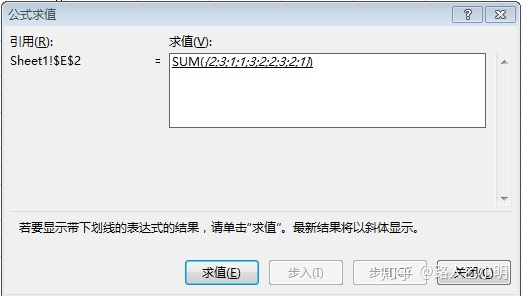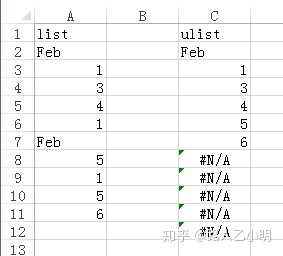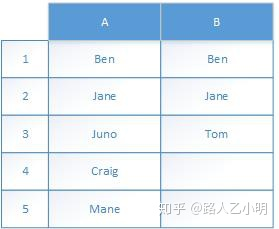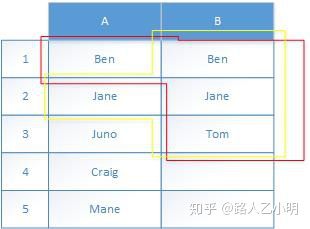# excel删除重复数据保留一条_[Excel]用公式删除重复项1. Excel可以用数据>删除重复项 把一组数据里面的重复单元格删掉, 但是这种操作要依靠手动, 不能自动更新, 所以如果你的数据来源发生更新的时候要自己重新做一遍, 容易出错
2. 要想能够自动完成数据>删除重复项 的功能, 用下面这个公式就可以了. 假设需要删除重复项的这组单元格是A2:A11, 删除重复项以后的唯一值列表放在C列, 在C2放置这个公式, CTRL+SHIFT+ENTER完成输入, 之后把单元格向下填充
=IFERROR(INDEX($A$2:$A$11,MATCH(0,COUNTIF($C$1:C1,$A$2:$A$11),0)),"")

• countif($C$1:C1,$A$2:$A$11)，这个记作countif(...)
• match(0, countif(...),0)，这个记作 match(...)
• index($A$2:$A$11, match(...)), 这个记作 index(...)
• match找不到的时候会报错，所以最外面再套一个iferror(index(...),"")

## 1. countif## 2. match和index

=MATCH(0,COUNTIF($C$1:C1,$A$2:$A$11),0)=countif(B:B,A1)=countif(B:B,A1:A2)

=INDEX($A$2:$A$11,MATCH(0,COUNTIF($C$1:C1,$A$2:$A$11),0))

11-30153611-2694
11-3098
09-261万+
09-253万+
06-043246
04-04165
08-203万+
09-031473
08-27753
06-131232
12-263968
12-2059
10-27
©️2020 CSDN 皮肤主题: 游动-白 设计师:白松林